# Zonal Statistics and Trial Plots extraction tools

## Introduction

Zonal Statistics and Trial Plot Extraction tools offer a quick and efficient way to digitize field trials and extract plot-level data for trials of different sizes and layouts. Zonal Statistics works with any type of imagery, both RGB and multispectral.

On a high level, the workflow includes following steps:

1. Create plot boundaries or custom zones
2. Calculate the statistics
3. Export the statistics

## Step 1. Create plot boundaries or custom zones

The first step is to create plot boundaries that will be used that will be used to outline the areas that statistics will be generated for. There are 4 different ways to create plot boundaries:

• Generate plots offers a semi-automated way of creating boundaries by providing the dimensions and gaps between the plots and visually matching them with the map.
• Auto-detect plots uses AI-algorithms to automatically detect the gaps between the plots when those are clearly visible.
• Custom zones allows creating plots or zones of any size and shape that are not necessarily limited to field trials and can be used to analyze selected parts of any field.
• Import SHP/KML can be used to import existing plots or zones from SHP or KML files. The files need to be in WGS84(EPSG:4326) coordinate system.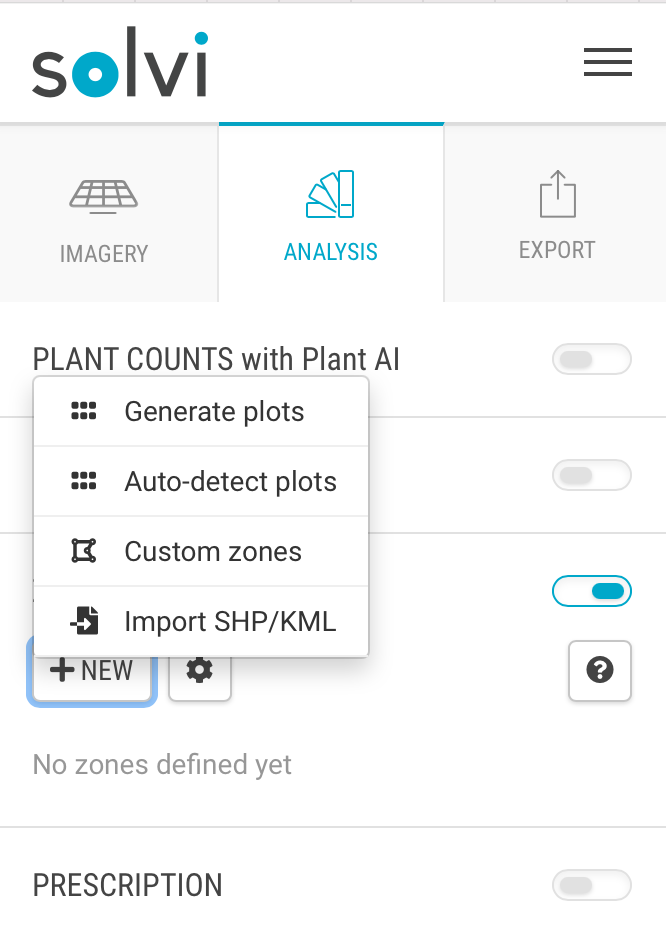#### Generate plots

This option suits well in the scenarios where the gaps between the plots are not very clear and the automated detection may not work well.

Click New -> Generate plots to start the workflow. A template grid with 5x5 plots will appear on the map.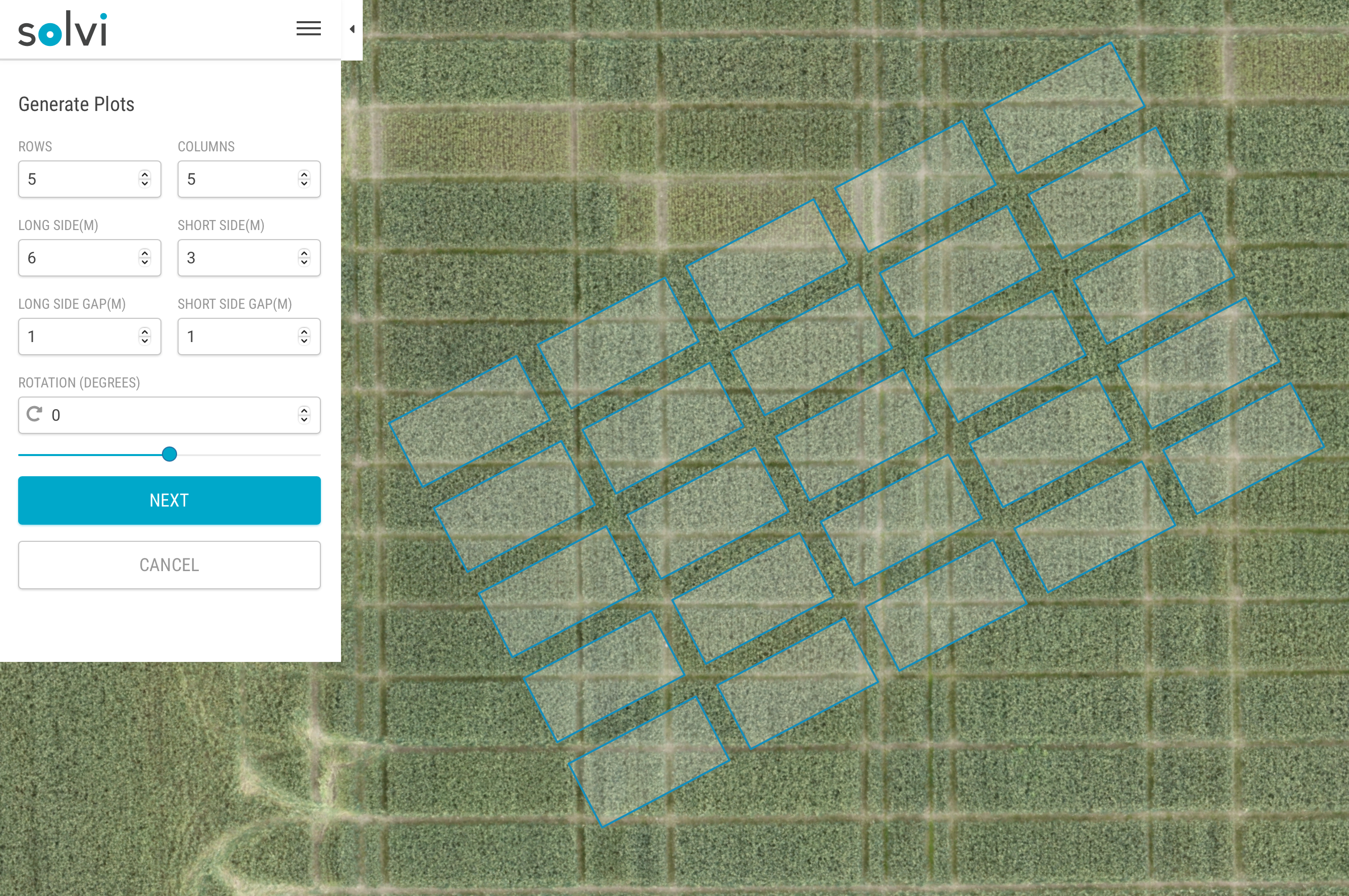Next step is to align the template with the imagery.

Drag the grid so that one of its corners matches the corner of the first plot in the imagery: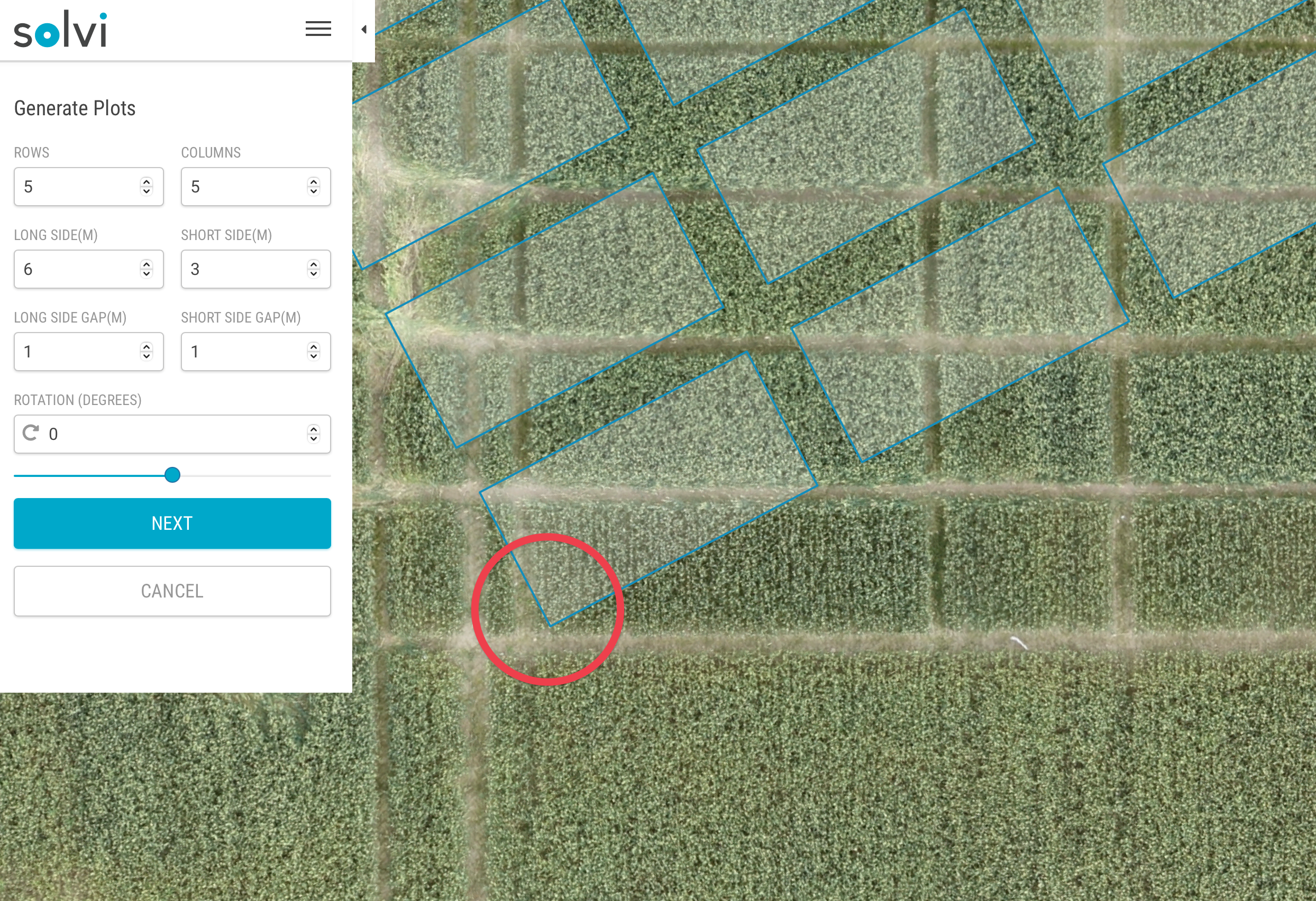After that, it's usually easiest to first align the Rotation of the grid, then the length of the long and short side of the plot and after - the gaps between the plots.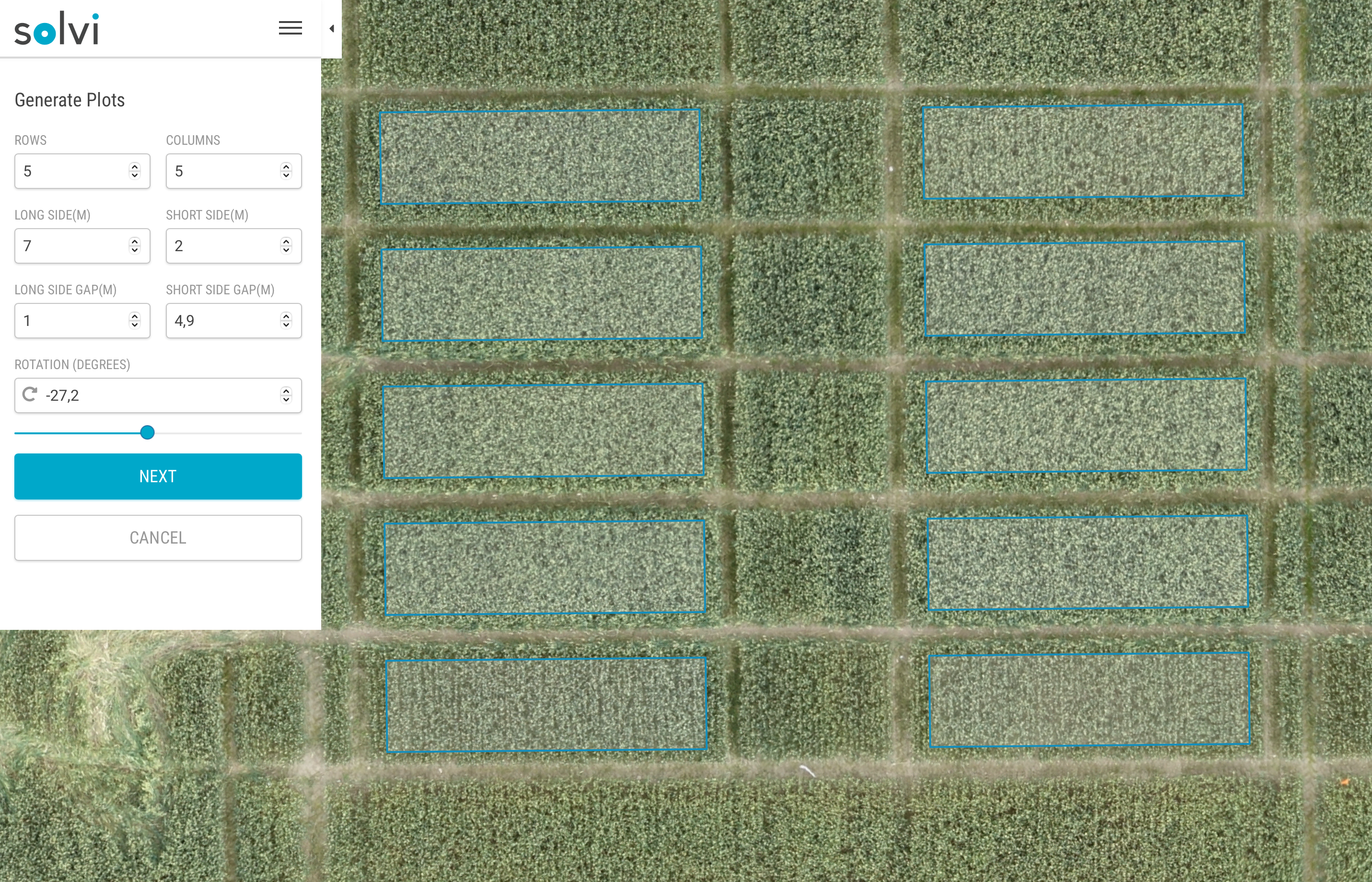Once the dimensions and gaps between the plots in the template match well with the background imagery, you can go ahead and adjust number of rows and columns so that the whole trial is covered with plots:Click "Next" to proceed to the step where you can specify plot numbering.Here you can specify from which corner the numbering should start, in which direction the numbering should go, what character to use as a separator between row and column numbers as well as how many digits to use.

Click "Save" to finalize this plot creation step.

#### Auto-detect plots

This method works best usually in cereal crops in mid to late growth stages when the gaps between the plots are clearly visible.

To auto-detect the plots, first outline approximately the trial area to exclude any other unwanted objects and click "Detect".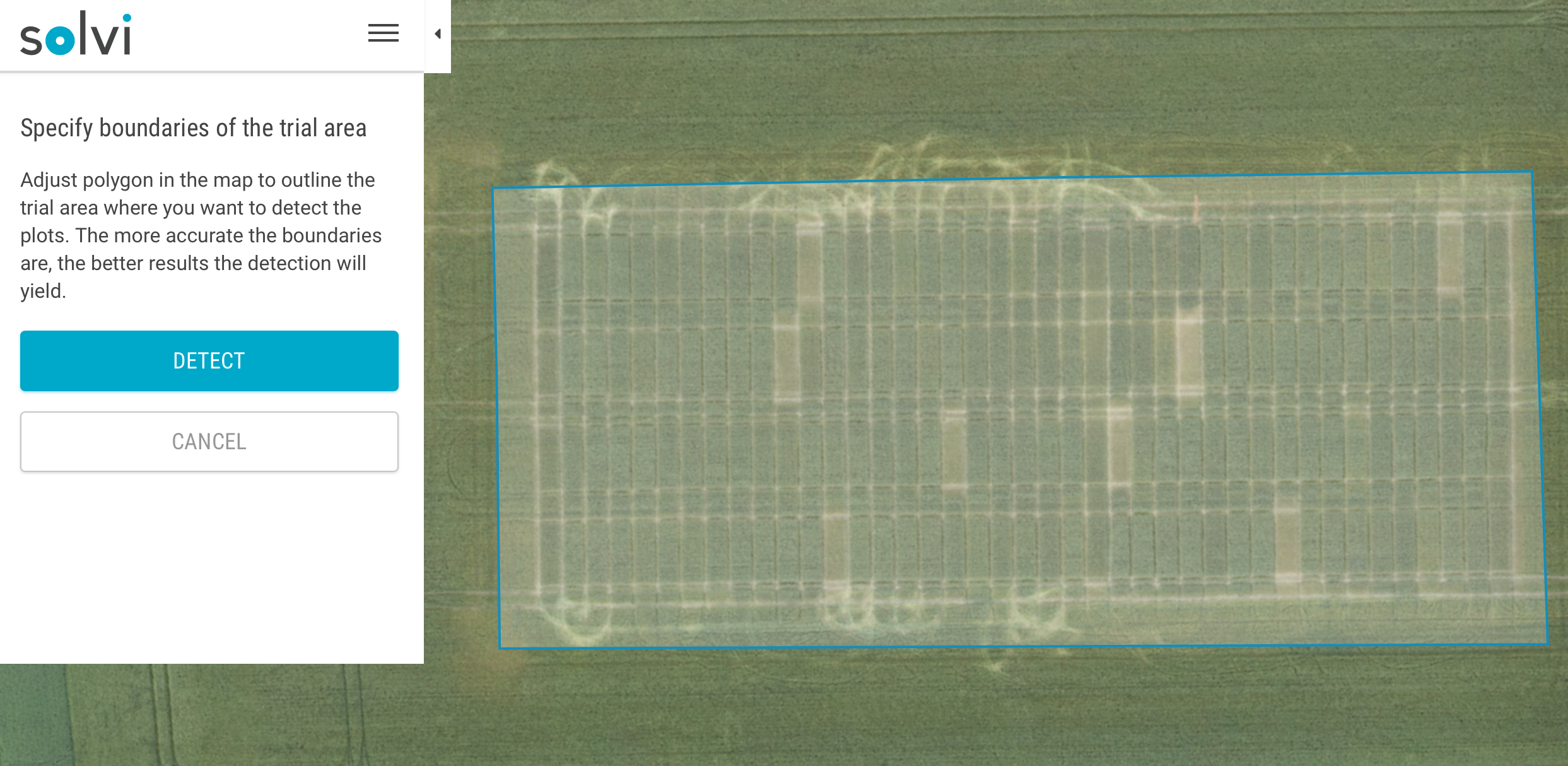Depending on the size of the trial and resolution of the imagery it may take anything from a few seconds to a few minutes for detection to finish. Once ready, you will see the detected plots outlined on the map: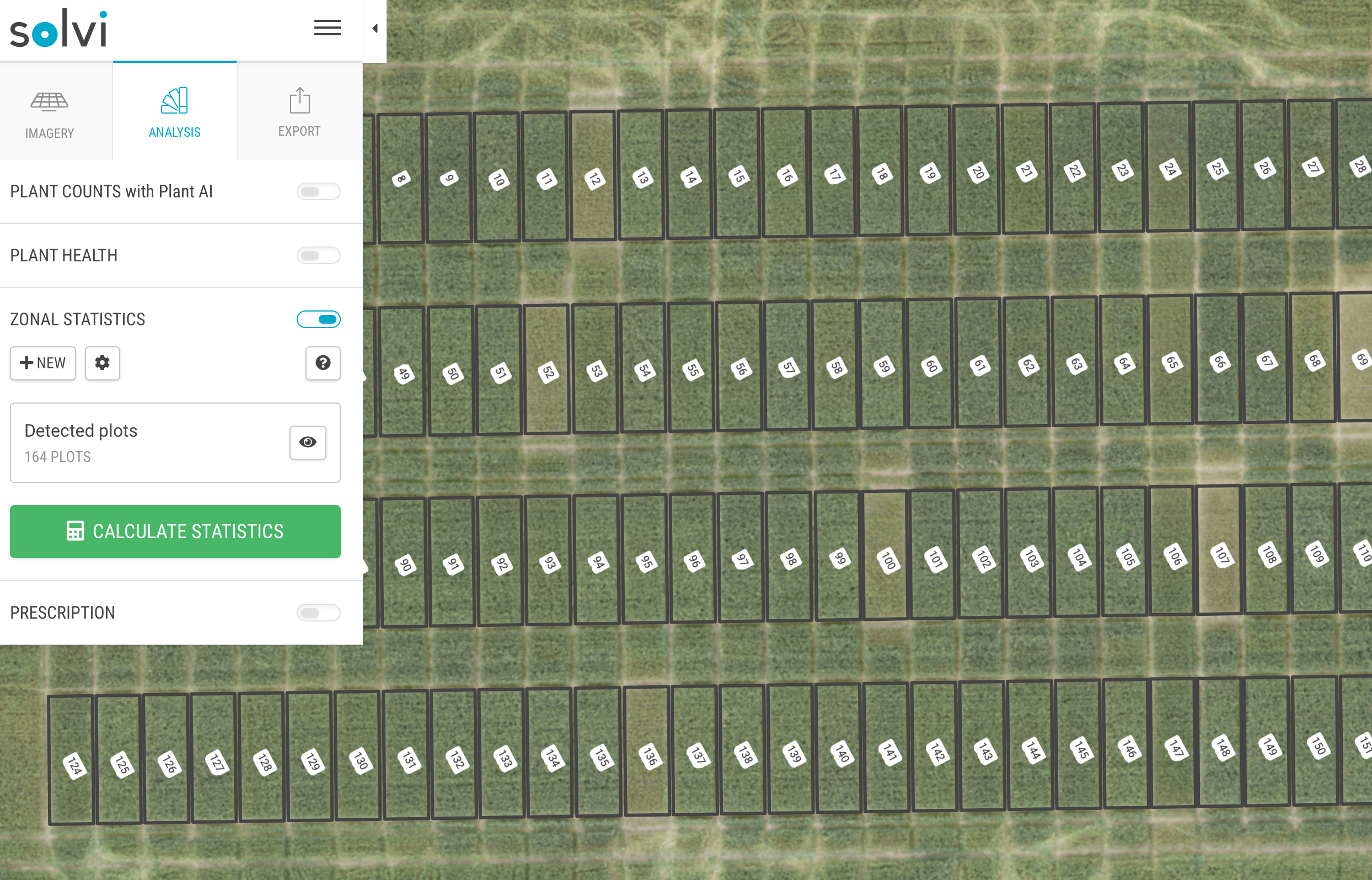#### Custom Zones

This option allows you to manually draw the plots or the polygons of custom shape and size using the drawing tools.

Once a few plots/zones are drawn, the copy/paste tools can be used to efficiently generate the plots for the whole field.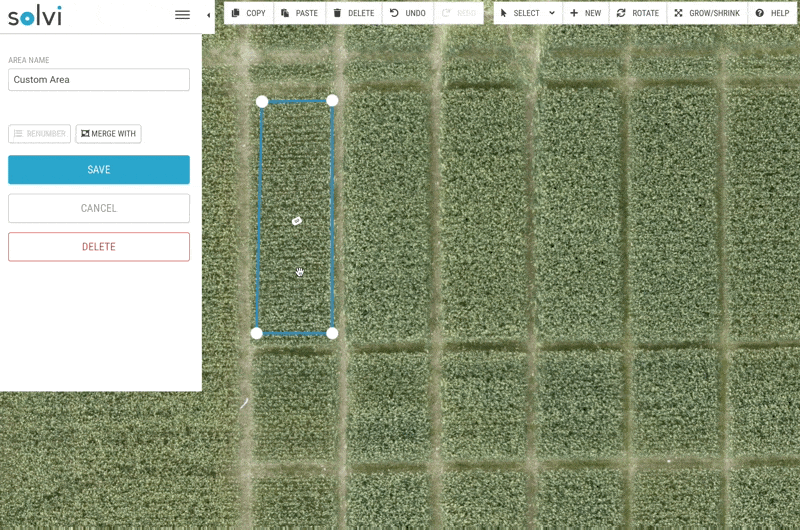#### Import SHP/KML

If you have previously created the plot boundaries in Solvi or other software, you can import them with this option.

The SHP or KML file needs to be in WGS84(EPSG:4326) coordinate system.

If you are importing the boundaries from the SHP-file, make sure to zip together .shp, .dbf, .shx and .prj files and choose the .zip file in order for the attributes like plot ids to follow along. If you only choose the .shp-file, only the geometries will be imported and the new plot ids will be generated automatically.

Step 2. Calculate and view statistics

Now that you have create the plot boundaries using one of the methods described above, the statistics can be easily calculated by clicking on the "Calculate Statistics"-button. The calculation may take a while depending on the number of plots and/or size of the dataset.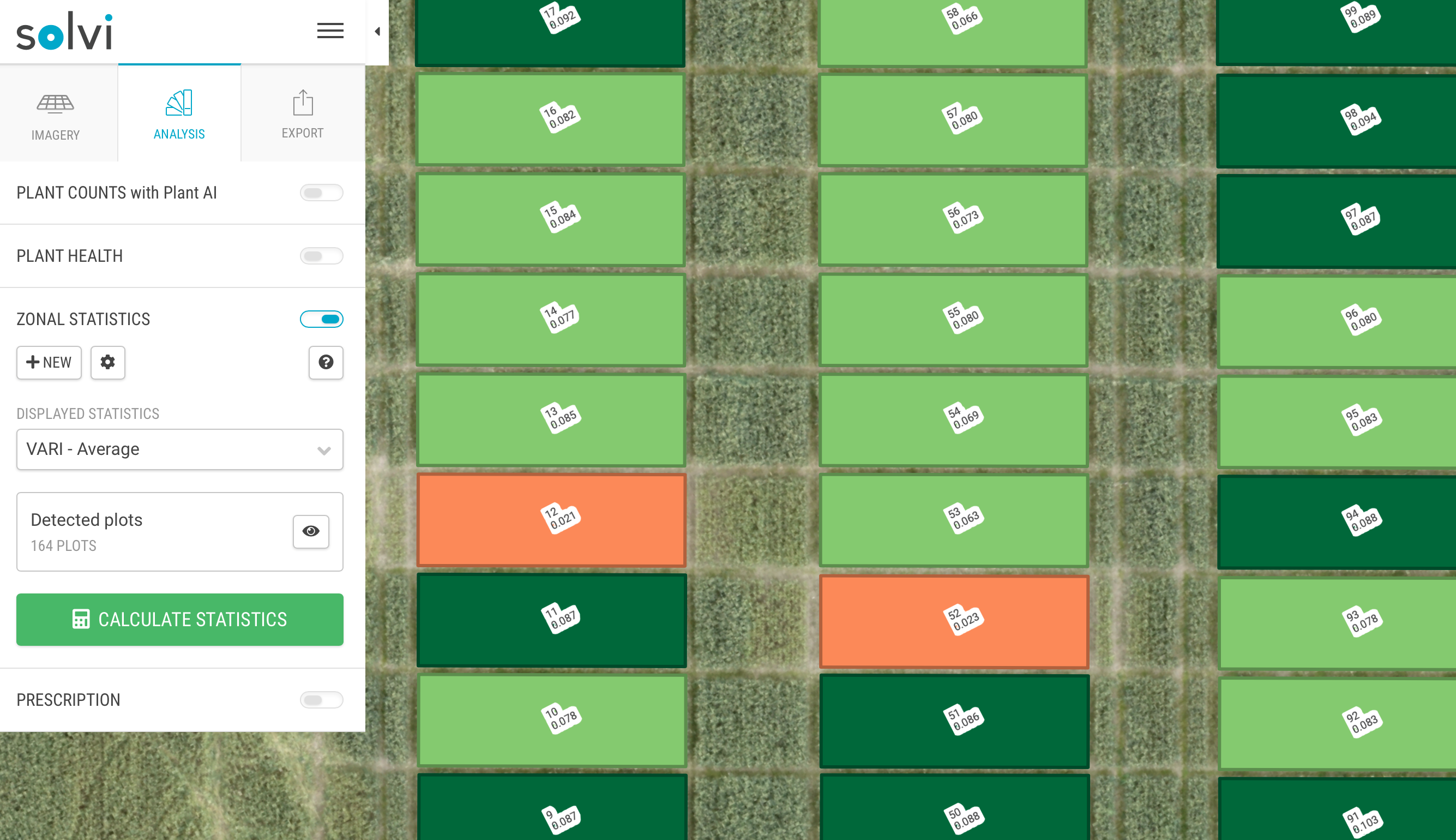Once ready, the statistic selected in the "Displayed Statistics" dropdown in the left pane will be displayed on top of each plot. In addition, all plots will be automatically categorized in 5 equal classes depending on the value of selected metric and assigned a color to make it easier to spot the differences. You can also click on any of the plots to see detailed statistics for that specific specific plot.

By default, following statistics will be calculated for each individual band of the imagery as well as for the currently selected vegetation index in the Plant Health tool:

• 25th and 75th percentile
• average value
• median value
• maximum value
• minimum value
• standard deviation

If you would like to calculate statistics for another vegetation index, simply switch to that index in the Plant Health Map and click again on "Calculate Statistics" to update the metrics. In general, this procedure needs to be run every time the new plots are created or the existing ones are modified.

In addition to statistics for raw bands and vegetation indices, the height and elevation statistics are also calculated if the dataset has an elevation map. The Elevation-statistics show the metrics described above for the raw values of the Elevation map, which usually is the number of meters above the sea level.

The Height statistics calculate the height of each plot by finding the lowest "base" point either right outside of the plot(statistics prefixed with the word "Outside")  or within the plot(statistics prefixed with the word "Inside") for trials in for example vegetables where it's common that the crops grow on raised beds and the height of the bed is thus not considered in calculations.

For the datasets with plant counts there will be additional metrics calculated such as number of plants in each plot, plant density(plants per hectare), and, if the plant counts also include size estimations - minimum, maximum, average and median plant diameter in each plot.

## Step 3. Export the results

All the metrics generated with Zonal Statistics tools can be exported in 2 formats:

• CSV-file that can be either viewed in Excel or accessed programmatically in languages like Python or R
• SHP-file for use in GIS software like QGIS or ArcGIS